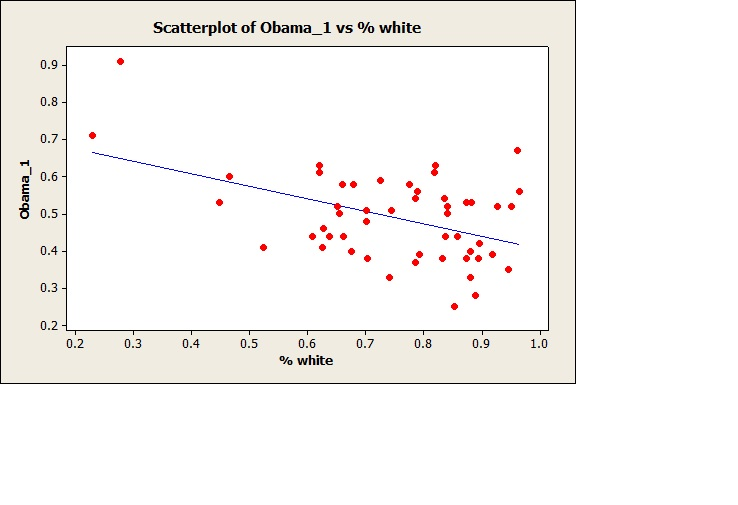# Do People Vote Their Pocket-Book or Clan? from Kim Zussman

November 18, 2012 |

2012 presidential election voting data by state was obtained from this source.

States were analyzed according to income ratio of top 5% to bottom 20% using this data.

Regressing the ratio (top 5%/bottom fifth) vs. fraction voting for Obama did not uncover a significant correlation:

Regression Analysis: 5%/bot fifth versus Obama

The regression equation is
5%/bot fifth = 10.5 + 3.11 Obama

Predictor    Coef  SE Coef     T      P
Constant   10.496    1.507 6.97 0.000
Obama       3.111    2.992  1.04  0.304

S = 2.50077   R-Sq = 2.2%   R-Sq(adj) = 0.2%

Based on this one cannot conclude that Obama's election results were correlated with income inequality. (NS also regressing vs % voting for Romney) How does this compare to state's racial demographics?

State racial demographic data was obtained from the 2000 census; extracting the ratio of those identifying as "non-hispanic/latino white" to total population (% white).

Using this data, regressed fraction voting for Obama vs % white by state:

Regression Analysis: Obama_1 versus % white

The regression equation is
Obama_1 = 0.740 - 0.334 % white

Predictor      Coef  SE Coef      T      P
Constant    0.73967  0.07075  10.45  0.000
% white    -0.33382  0.09241  -3.61  0.001

S = 0.106100   R-Sq = 21.0%   R-Sq(adj) = 19.4%

In terms of state populations, there was a significant negative correlation between % voting for Obama and % white. A similar opposite) positive correlation was found for % voting for Romney (not shown).